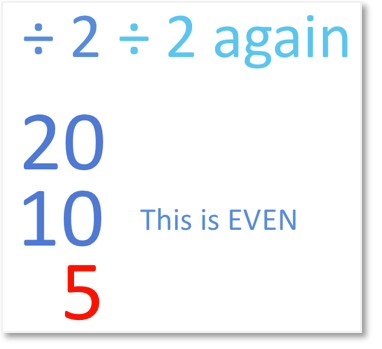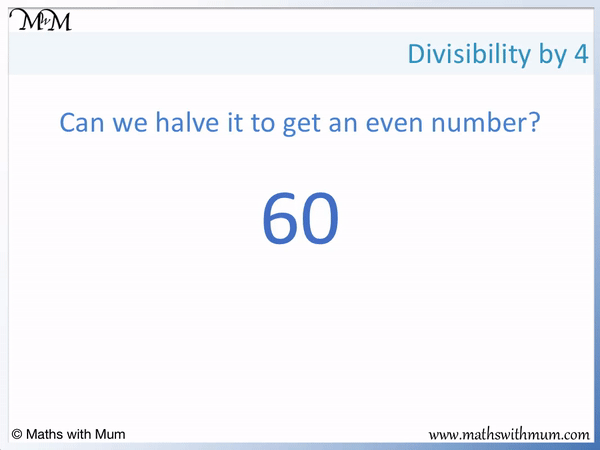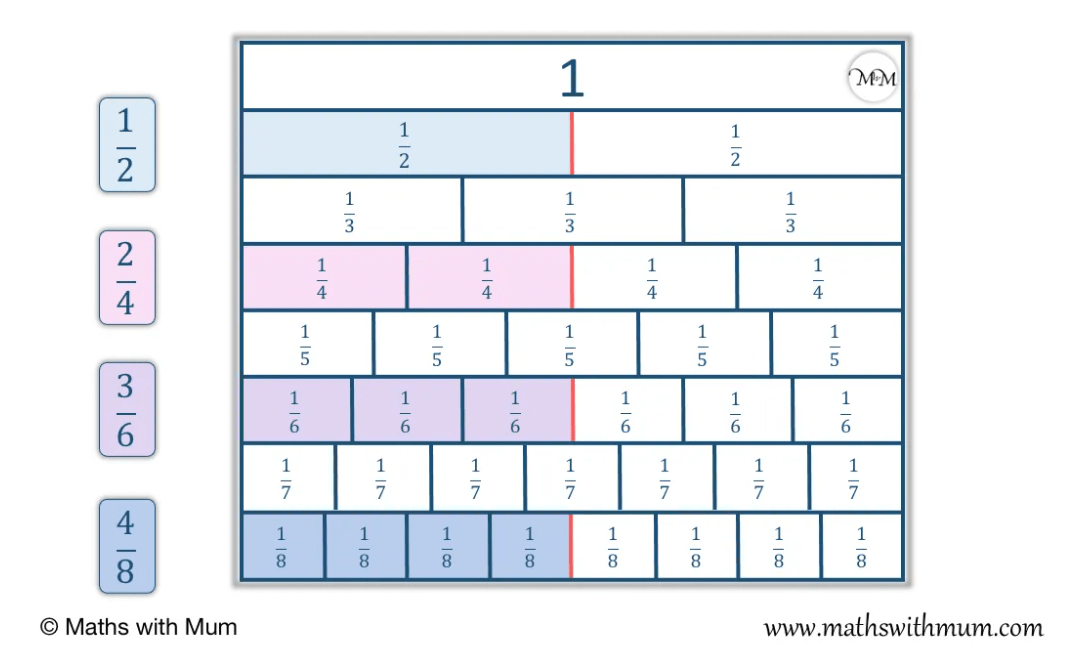# How to Tell if a Number is Divisible by 4

How to Tell if a Number is Divisible by 4• A number is divisible by 4 if its last two digits are divisible by 4.
• A number is also divisible by 4 if it can be halved and then halved again to leave a whole number.
• To test if a large number is a multiple of 4, we only need to look at the last 2 digits.
• The last two digits of 732 are 32.
• 32 is divisible by 4 because 32 = 4 × 8.
• We can also halve 32 to make 16 and halve 16 to make 8. 32 can be halved and halved again so it is a multiple of 4.
• 32 is divisible by 4 and therefore 732 is also divisible by 4.
• The rule works because 4 divides exactly into 100. This means that all multiples of 100 are divisible by 4.
• 732 = 700 + 32. 700 is divisible by 4 so we only need to check the 32.
A number is divisible by four if its last two digits are divisible by 4.• A number is divisible by 4 if its last two digits are divisible by 4.
• The last two digits of 44,422 are 22.
• 22 is not a multiple of 4.
• We know this because half of 22 is 11 and then half of 11 is 5.5, which is not a whole number.
• 22 is not a multiple of 4 and so, 44,422 is not a multiple of 4 either.#### The Halve and Halve Again Strategy to Divide by 4# Divisibility by 4

## How to Tell if a Number is Divisible by 4

To decide if a number is divisible by 4, follow these steps:

1. Look at the last two digits in the tens and ones columns of the number.
2. If this 2-digit number is divisible by 4, then the original number is divisible by 4.
3. All 2-digit numbers are divisible by 4 if they can be halved and halved again to give a whole number.

If a number is divisible by 4, this means that it is a multiple of 4. A number that is divisible by 4 is in the 4 times table and can be divided exactly by 4 leaving no remainder.

A number is divisible by 4 if its last 2 digits are divisible by 4. There is no need to look at the preceding digits. All numbers are divisible by 4 if they can be halved and halved again to give a whole number.

For example, we will test if 732 is divisible by 4.The first step is to look at the last 2 digits of the number.

The last two digits of 732 are 32.

The next step if to decide if the last 2 digits are divisible by 4.

32 can be halved and then halved again to give a whole number. 32 ÷ 2 = 16 and then 16 ÷ 2 = 8. 32 is a multiple of 4 because it is 4 × 8.

32 is divisible by 4 and so, 732 is divisible by 4.

This means that 732 can be divided exactly by 4.

732 divided by 4 is 183.

The divisibility by 4 rule only tells us if a number is divisible by 4 but it does not tell us the answer to the division.

Here is an example of using the divisibility by 4 rule to prove that a number is not divisible by 4.

We have 44,422.Looking at the last two digits of 44,422, we have 22.

22 is not a multiple of 4 and so, the number 44,422 is not a multiple of 4 either.

We know that 22 is not a multiple of 4 because it cannot be halved and halved again to leave a whole number.

Half of 22 is 11 and half of 11 is 5.5.

Here is another example of using the rule to test for divisibility by 4.

Here is 3740.The last two digits are 40.

40 is a multiple of 4 and so, 3740 is too.

We can see that 40 can be halved and halved again to make 10. 40 is 10 lots of 4.

## Halve and Halve Again Strategy

The halve then halve again strategy is a method used to divide larger numbers by 4. Dividing by 4 is the same as dividing by 2 and then dividing by 2 again. This method is used to break down more complicated divisions into more manageable steps.

For example to divide 20 by 5, we halve 20 and then halve it again.

Half of 20 is 10. 10 is an even number and we can halve it again.

Half of 10 is 5. Therefore 20 ÷ 4 = 5.Although the halve and halve again strategy has 2 steps, they are generally easier to manage than simply dividing a number by 4. This means that this strategy is useful for dividing by 4 mentally.

A simple way to test if a number is divisible by 4, we simply need to be able to halve it and halve it again. If after halving once, the number is even, then it can be halved again. If a number gives an even number when halved then it is divisible by 4.

Here is 60 ÷ 4.Half of 60 is 30. We can see that 30 ends in a 0 and so, it is even.

60 must be divisible by 4 because it gives an even number when halved.

Half of 30 is 15 and so, 4 × 15 = 60. 4 divides into 60 fifteen times.

## List of Numbers Divisible by 4

There are 25 numbers between 0 and 100 that are divisible by 4:

4, 8, 12, 16, 20, 24, 28, 32, 36, 40, 44, 48, 52, 56, 60, 64, 68, 72, 76, 80, 84, 88, 92, 96, 100.

Because 100 ends in a 0 the pattern repeats again for the next 100 numbers.

We have:

104, 108, 112, 116, 120, 124, 128, 132, 136, 140, 144, 148, 152, 156, 160, 164, 168, 172, 176, 180, 184, 188, 192, 196, 200.

There are 25 multiples of 4 for each hundred numbers. If you know the first 25 two-digit multiples of 4, then these same two digits are in every multiple of 4.Now try our lesson on How to Find Equivalent Fractions where we learn what an equivalent fraction is and how to calculate them.